CAT  >  Test Level 3: Bar Graph

# Test Level 3: Bar Graph

Test Description

## 20 Questions MCQ Test Level-wise Tests for CAT | Test Level 3: Bar Graph

Test Level 3: Bar Graph for CAT 2023 is part of Level-wise Tests for CAT preparation. The Test Level 3: Bar Graph questions and answers have been prepared according to the CAT exam syllabus.The Test Level 3: Bar Graph MCQs are made for CAT 2023 Exam. Find important definitions, questions, notes, meanings, examples, exercises, MCQs and online tests for Test Level 3: Bar Graph below.
Solutions of Test Level 3: Bar Graph questions in English are available as part of our Level-wise Tests for CAT for CAT & Test Level 3: Bar Graph solutions in Hindi for Level-wise Tests for CAT course. Download more important topics, notes, lectures and mock test series for CAT Exam by signing up for free. Attempt Test Level 3: Bar Graph | 20 questions in 40 minutes | Mock test for CAT preparation | Free important questions MCQ to study Level-wise Tests for CAT for CAT Exam | Download free PDF with solutions
 1 Crore+ students have signed up on EduRev. Have you?
Test Level 3: Bar Graph - Question 1

### Directions: Answers the question based on the given information. A management institute was established on 1st January, 2000, with 3, 4, 5 and 6 faculty members respectively in Marketing, Organisational Behaviour (OB), Finance, and Operations Management (OM) departments to start with. No faculty member retired or joined the institute in the first three months of the year 2000. In the next four years, the institute recruited one faculty member in each of the four departments. All these new faculty members, who joined the institute subsequently over the years, were 25 years old at the time of their joining the institute. All of them joined the institute on 1st April. During these four years, one of the faculty members retired at the age of 60. The following bar chart gives the department-wise average age (in terms of number of completed years) of faculty members as on April 1 of 2000, 2001, 2002 and 2003.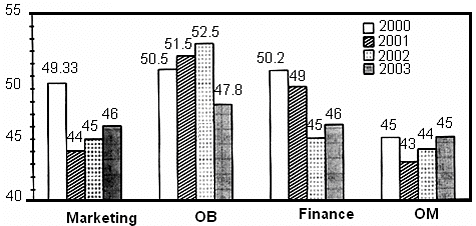Q. What was the age of the new faculty member who joined the OM department on 1st April, 2003?

Detailed Solution for Test Level 3: Bar Graph - Question 1

As there is a dip in the average age in OM in 2001, and because of the fact that there is only 1 dip in the OM data set, in the OM department, a new person joined in 2001 at the age of 25 years.
Therefore, the age of the new faculty member who joined the OM department on 1st April, 2003, was 27 years.

Test Level 3: Bar Graph - Question 2

### Directions: Answers the question based on the given information. A management institute was established on 1st January, 2000, with 3, 4, 5 and 6 faculty members respectively in Marketing, Organisational Behaviour (OB), Finance, and Operations Management (OM) departments to start with. No faculty member retired or joined the institute in the first three months of the year 2000. In the next four years, the institute recruited one faculty member in each of the four departments. All these new faculty members, who joined the institute subsequently over the years, were 25 years old at the time of their joining the institute. All of them joined the institute on 1st April. During these four years, one of the faculty members retired at the age of 60. The following bar chart gives the department-wise average age (in terms of number of completed years) of faculty members as on April 1 of 2000, 2001, 2002 and 2003.Q. Professors Naresh and Devesh, two faculty members of Marketing who have been with the institute since its inception, share their birthdays, which fall on 20th November. One was born in 1947 and the other in 1950. On 1st April, 2005, what was the approximate age of the third faculty member, who has been in the same area since inception?

Detailed Solution for Test Level 3: Bar Graph - Question 2

In the table, 'n' represents the number of faculty members in different areas and 'a' represents the average age of faculty members.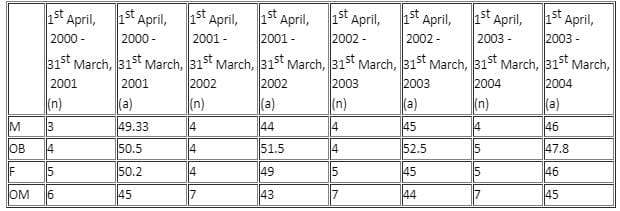Sum of ages of Naresh and Devesh in 2000 = (52 + 49) years = 101 years
In 2000, age of the third faculty member = (49.33 × 3 - 101) years = 47 years
In 2005, age of the third faculty member = (47 + 5) years = 52 years

Test Level 3: Bar Graph - Question 3

### Directions: Answers the question based on the given information. A management institute was established on 1st January, 2000, with 3, 4, 5 and 6 faculty members respectively in Marketing, Organisational Behaviour (OB), Finance, and Operations Management (OM) departments to start with. No faculty member retired or joined the institute in the first three months of the year 2000. In the next four years, the institute recruited one faculty member in each of the four departments. All these new faculty members, who joined the institute subsequently over the years, were 25 years old at the time of their joining the institute. All of them joined the institute on 1st April. During these four years, one of the faculty members retired at the age of 60. The following bar chart gives the department-wise average age (in terms of number of completed years) of faculty members as on April 1 of 2000, 2001, 2002 and 2003.Q. From which department did the faculty member retire?

Detailed Solution for Test Level 3: Bar Graph - Question 3

In the table, 'n' represents the number of faculty members in different departments and 'a' represents the average age of faculty members.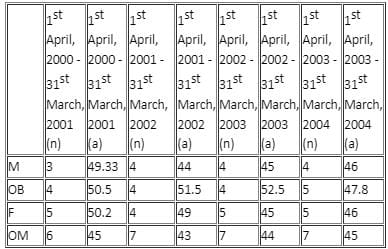It is given that 1 person was recruited in each of the departments. This is the key sentence.
There is a dip in average age twice only in Finance. Whenever a person joins or leaves, the average age would be disturbed from its normal increasing value of 1 every year.
Now, as 2 persons have not joined the same department, it must only be in Finance that a person retired in 1 year and another member joined in another year. Because of the fact that all departments have 1 dip in their average age, and Finance has 2, the possibility that the person who retired at 60 years of age and a new faculty member joined in the same department in the same year is ruled out.
Furthermore, the normal trend in average age in each department is an increase of 1, which would be the normal behaviour if no person joins in or leaves, unless and until the average age of persons who leave and join in the same year is exactly the same.
Furthermore, as the trend is decrease in age and it is happening only once in each of the departments except Finance, the possibility that the average age would increase when a person joins is ruled out as only 1 person joins in the entire period in the data set.

Test Level 3: Bar Graph - Question 4

Directions: Answers the question based on the given information.

A management institute was established on 1st January, 2000, with 3, 4, 5 and 6 faculty members respectively in Marketing, Organisational Behaviour (OB), Finance, and Operations Management (OM) departments to start with. No faculty member retired or joined the institute in the first three months of the year 2000. In the next four years, the institute recruited one faculty member in each of the four departments. All these new faculty members, who joined the institute subsequently over the years, were 25 years old at the time of their joining the institute. All of them joined the institute on 1st April. During these four years, one of the faculty members retired at the age of 60. The following bar chart gives the department-wise average age (in terms of number of completed years) of faculty members as on April 1 of 2000, 2001, 2002 and 2003.Q. In which year did the new faculty member join the Finance department?

Detailed Solution for Test Level 3: Bar Graph - Question 4

It is given that 1 person was recruited in each of the departments. This is the key sentence.
There is a dip in average age twice only in Finance. Whenever a person joins or leaves, the average age would be disturbed from its normal increasing value of 1 every year.
Now, as 2 persons have not joined the same department, it must only be in Finance that a person retired in 1 year and another member joined in another year. Because of the fact that all departments have 1 dip in their average age, and Finance has 2, the possibility that the person who retired at 60 years of age and a new faculty member joined in the same year is ruled out.
Furthermore, the normal trend in average age in each department is an increase of 1, which would be the normal behaviour if no person joins in or leaves, unless and until the average age of persons who leave and join in the same year is exactly the same.
Furthermore, as the trend is decrease in age and it is happening only once in each of the departments except Finance, the possibility that the average age would increase when a person joins is ruled out as only 1 person joins in the entire period in the data set.
Now, let us assume that the person who retired at the age of 60 years from the Finance department, retired in the period 2nd April, 2000 - 31st March, 2001, both dates inclusive. So, the average age of the faculty members as on 1st April, 2001, should be (50.2 × 5 + 5 - 60)/4) years = 49 years, which matches perfectly with the data given.
Thus, the person that retired must have done so in the period given.
Now, there is a dip in the average age of faculty of the Finance department in the year 2002.
So, let us now evaluate if it matches with the data given.
If a person of 25 years of age joins the Finance department on 1st April, 2002, then the average age of the faculty members on 1st April, 2002 is ((49 × 4 + 4 + 25)/5) years = 45 years, which matches perfectly with the given data.
Thus, the person who joined the Finance department did so in the year 2002.
Hence, answer option 3 is correct.

Test Level 3: Bar Graph - Question 5

Directions: Answer the question on the basis of bar graphs given below.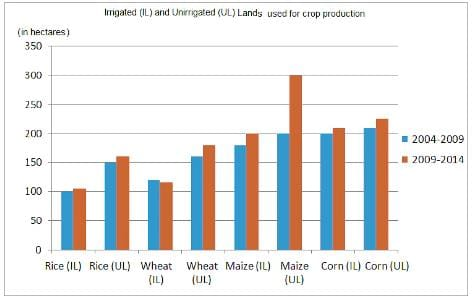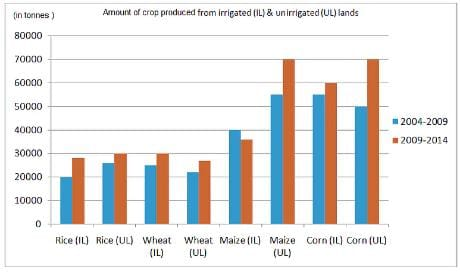Q. Between the two given periods, the production in tonnes from irrigated land as a percentage of total production (irrigated land + unirrigated land for a particular crop) increased for

Detailed Solution for Test Level 3: Bar Graph - Question 5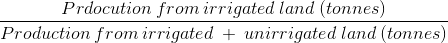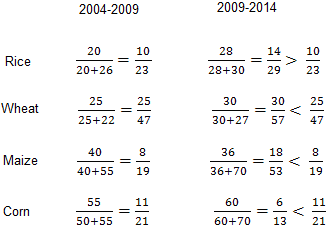Hence, option (1) is correct.

Test Level 3: Bar Graph - Question 6

Directions: Answer the question on the basis of bar graphs given below.Q. During 2009-2014, for which of the following crops is the production in tonnes per hectare more for irrigated land than for unirrigated land?

Detailed Solution for Test Level 3: Bar Graph - Question 6

Production per hectare ('000 tonnes/hectare)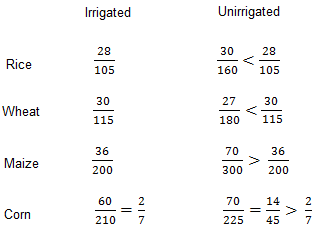Hence, option (3) is correct.

Test Level 3: Bar Graph - Question 7

Directions: Answer the question on the basis of bar graphs given below.Q. During 2004 - 2009, the difference between rice and wheat production from irrigated land and rice and wheat production from unirrigated land is

Detailed Solution for Test Level 3: Bar Graph - Question 7

Irrigated land         Unirrigated land
Rice       20000 tonnes          26000 tonnes
Wheat    25000 tonnes        22000 tonnes
Total       45000 tonnes        48000 tonnes
Therefore, Difference = 48000 tonnes – 45000 tonnes = 3000 tonnes
Hence, option (3) is correct.

Test Level 3: Bar Graph - Question 8

Directions: Answer the question on the basis of bar graphs given below.Q. During 2009 - 2014, maize production in tonnes per hectare of irrigated land as compared to that in 2004 - 2009 declined by

Detailed Solution for Test Level 3: Bar Graph - Question 8

Maize production per hectare of irrigated land.
2009-2014 =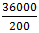= 180 tonnes/hectares
2004-2009 =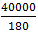= 222 tonnes/hectares
Therefore, Decline = 222 - 180 = 42 tonnes/hectares.
Hence, option (2) is correct.

Test Level 3: Bar Graph - Question 9

Indian Kabbadi team captain Ajay Thakur scored points against 6 different teams in Pro Kabbadi 2017 and Pro Kabbadi 2018. The bar charts given below depict the number of points scored by Ajay against the opponent as a percentage of total points scored in that Pro Kabbadi series. It is also given that the ratio of points scored by Ajay against Bengal Warriors in the Pro Kabbadi 2017 and Pro Kabbadi 2018 is 2 : 1, whereas the points scored against Bengaluru Bulls is the same in both Pro Kabbadi series.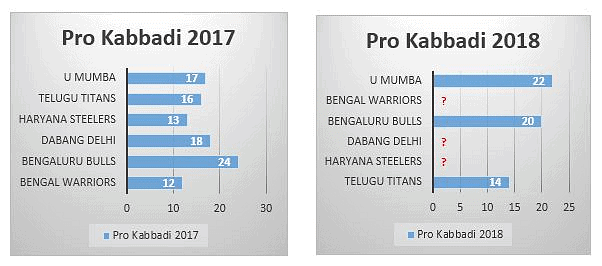Q. If it is given that Ajay scored minimum possible points in Pro Kabbadi 2018, then what is the maximum difference in number of points scored by him against Haryana Steelers in both the Pro Kabbadi series?

Detailed Solution for Test Level 3: Bar Graph - Question 9

Suppose 100p and 100q are the total number of points scored in Pro Kabbadi 2017 and 2018, respectively.
So, the points scored by Ajay against Bengaluru Bulls in the year 2017 = 0.24 × 100p = 24p
The points scored by Ajay against Bengaluru Bulls in the year 2018 = 0.20 × 100q = 20q
Therefore,
20q = 24p or q = 1.2p
Now, we represent the number of points against each opponent in both Pro Kabbadi series in terms of p.
The number of points scored by Ajay against Bengal Warriors in 2017 = 12p
The ratio is given as 2 : 1.
Therefore, points scored by Ajay against Bengal Warriors in 2018 = 6p
That is 5 percent of the total number of points scored by Ajay in 2018.
But, we do not know percentage of points scored by Ajay against Dabang Delhi and Haryana Steelers in 2018.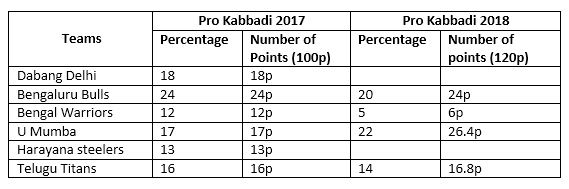Let a be the percentage of points scored by Ajay against Dabang Delhi in 2018.
Then, the number of points scored by Ajay against Dabang Delhi = 1.2p × a = (6a/5)p
Now, the remaining table can be filled.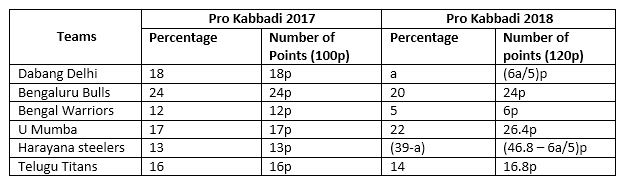So, Ajay scored 26.4p and 16.8q points against U Mumba and Telugu Titans in 2018.
Hence, we can say that p is a multiple 5.
The total number of points scored by Ajay in 2018 will be minimum when p = 5
Total number of points against Haryana Steelers in 2017 = 13 × 5 = 65
Hence, the difference in number of points scored against Harayana Steelers in both Pro Kabbadi series
= |(234 - 6a) - 65| = 169 - 6a
The difference will be maximum, if a = 0
Therefore, maximum difference = 169

Test Level 3: Bar Graph - Question 10

Indian Kabbadi team captain Ajay Thakur scored points against 6 different teams in Pro Kabbadi 2017 and Pro Kabbadi 2018. The bar charts given below depict the number of points scored by Ajay against the opponent as a percentage of total points scored in that Pro Kabbadi series. It is also given that the ratio of points scored by Ajay against Bengal Warriors in the Pro Kabbadi 2017 and Pro Kabbadi 2018 is 2 : 1, whereas the points scored against Bengaluru Bulls is the same in both Pro Kabbadi series.Q. In Pro Kabbadi 2018, the sum of points scored against Dabang Delhi and Harayana Steelers as a percentage of total points is

Detailed Solution for Test Level 3: Bar Graph - Question 10

Suppose 100p and 100q are the total number of points scored in Pro Kabbadi 2017 and 2018, respectively.
So, the points scored by Ajay against Bengaluru Bulls in the year 2017 = 0.24 × 100p = 24p
The points scored by Ajay against Bengaluru Bulls in the year 2018 = 0.20 × 100q = 20q
Therefore,
20q = 24p or q = 1.2p
Now, we represent the number of points against each opponent in both Pro Kabbadi series in terms of p.
The number of points scored by Ajay against Bengal Warriors in 2017 = 12p
The ratio is given as 2 : 1.
Therefore, points scored by Ajay against Bengal Warriors in 2018 = 6p
That is 5 percent of the total number of points scored by Ajay in 2018.
But, we do not know percentage of points scored by Ajay against Dabang Delhi and Haryana Steelers in 2018.Let a be the percentage of points scored by Ajay against Dabang Delhi in 2018.
Then, the number of points scored by Ajay against Dabang Delhi = 1.2p × a = (6a/5)p
Now, the remaining table can be filled.From the above table, the sum of points scored against Dabang Delhi and Harayana Steelers in Pro Kabbadi 2018 as a percentage of total points scored = 39 – a + a = 39%

Test Level 3: Bar Graph - Question 11

Indian Kabbadi team captain Ajay Thakur scored points against 6 different teams in Pro Kabbadi 2017 and Pro Kabbadi 2018. The bar charts given below depict the number of points scored by Ajay against the opponent as a percentage of total points scored in that Pro Kabbadi series. It is also given that the ratio of points scored by Ajay against Bengal Warriors in the Pro Kabbadi 2017 and Pro Kabbadi 2018 is 2 : 1, whereas the points scored against Bengaluru Bulls is the same in both Pro Kabbadi series.Q. If the difference between points scored by Ajay against Dabang Delhi in both 2017 and 2018 is zero, then by what percentage is the number of points scored by Ajay against U Mumba, Haryana Steelers and Telugu Titans combined in Pro Kabbadi 2018 higher than in Pro Kabbadi 2017 against the same teams?

Detailed Solution for Test Level 3: Bar Graph - Question 11

Suppose 100p and 100q are the total number of points scored in Pro Kabbadi 2017 and 2018, respectively.
So, the points scored by Ajay against Bengaluru Bulls in the year 2017 = 0.24 × 100p = 24p
The points scored by Ajay against Bengaluru Bulls in the year 2018 = 0.20 × 100q = 20q
Therefore,
20q = 24p or q = 1.2p
Now, we represent the number of points against each opponent in both Pro Kabbadi series in terms of p.
The number of points scored by Ajay against Bengal Warriors in 2017 = 12p
The ratio is given as 2 : 1.
Therefore, points scored by Ajay against Bengal Warriors in 2018 = 6p
That is 5 percent of the total number of points scored by Ajay in 2018.
But, we do not know percentage of points scored by Ajay against Dabang Delhi and Haryana Steelers in 2018.Let a be the percentage of points scored by Ajay against Dabang Delhi in 2018.
Then, the number of points scored by Ajay against Dabang Delhi = 1.2p × a = (6a/5)p
Now, the remaining table can be filled.It is given that the difference in number of points scored by Ajay against Dabang Delhi in both Pro Kabbadi series is zero.
i.e. 18p = (6a/5)p
a = 15
The number of points scored by Ajay against U Mumba, Haryana Steelers and Telugu Titans combined in the Pro Kabbadi 2018 = 26.4p + 28.8p + 16.8p = 72p
The number of points scored by Ajay against U Mumba, Haryana Steelers and Telugu Titans combined in the Pro Kabbadi 2017 = 17p + 13p + 16p = 46p
Hence, the required percentage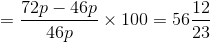Test Level 3: Bar Graph - Question 12

Indian Kabbadi team captain Ajay Thakur scored points against 6 different teams in Pro Kabbadi 2017 and Pro Kabbadi 2018. The bar charts given below depict the number of points scored by Ajay against the opponent as a percentage of total points scored in that Pro Kabbadi series. It is also given that the ratio of points scored by Ajay against Bengal Warriors in the Pro Kabbadi 2017 and Pro Kabbadi 2018 is 2 : 1, whereas the points scored against Bengaluru Bulls is the same in both Pro Kabbadi series.Q. In Pro Kabbadi 2018, the percentage of points scored against Dabang Delhi with respect to the total points scored is

Detailed Solution for Test Level 3: Bar Graph - Question 12

Suppose 100p and 100q are the total number of points scored in Pro Kabbadi 2017 and 2018, respectively.
So, the points scored by Ajay against Bengaluru Bulls in the year 2017 = 0.24 × 100p = 24p
The points scored by Ajay against Bengaluru Bulls in the year 2018 = 0.20 × 100q = 20q
Therefore,
20q = 24p or q = 1.2p
Now, we represent the number of points against each opponent in both Pro Kabbadi series in terms of p.
The number of points scored by Ajay against Bengal Warriors in 2017 = 12p
The ratio is given as 2 : 1.
Therefore, points scored by Ajay against Bengal Warriors in 2018 = 6p
That is 5 percent of the total number of points scored by Ajay in 2018.
But, we do not know percentage of points scored by Ajay against Dabang Delhi and Haryana Steelers in 2018.Let a be the percentage of points scored by Ajay against Dabang Delhi in 2018.
Then, number of points scored by Ajay against Dabang Delhi = 1.2p × a = (6a/5)p
Now, the remaining table can be filled.We cannot determine points scored against Dabang Delhi as a percentage of total points scored in Pro Kabbadi 2018.

Test Level 3: Bar Graph - Question 13

Directions: The given questions are based on the following bar graphs giving the number of students in different branches in two engineering colleges for the years 1996 - 1997 and 1997 - 98: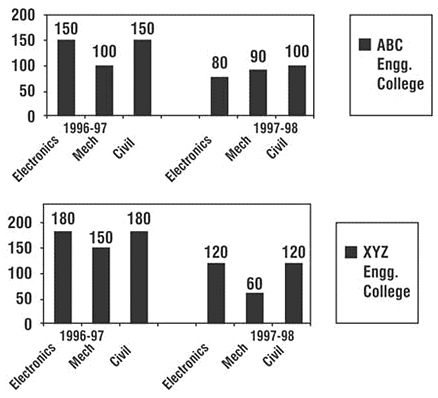Q. If 60 students from electronics department and 60 students from mechanical department of XYZ Engineering College shift to ABC Engineering College in the year 1996 - 1997, then the percentage of electronics students in XYZ Engineering College to the total number of students in the college in 1996 - 1997 will be

Detailed Solution for Test Level 3: Bar Graph - Question 13

otal number of students in XYZ
Engineering College in 1996 - 97, if 60
students of electronics department and 60
students of mechanical department of the
college shift to ABC Engineering College = 180 + 150 + 180 - 60 - 60 = 390
Number of students of electronics department left in XYZ Engineering College = 120
Percent =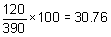%, i.e. 31% (approx.)

Test Level 3: Bar Graph - Question 14

Directions: The given questions are based on the following bar graphs giving the number of students in different branches in two engineering colleges for the years 1996 - 1997 and 1997 - 98:Q. The number of students in XYZ engineering college for the years 1996 - 97 and 1997 - 98, put together, exceeds the number of students in ABC Engineering College, put together, for the same period by

Detailed Solution for Test Level 3: Bar Graph - Question 14

The number of students of ABC Engineering
College in (1996 - 97 and 1997 - 98) = 150 + 100 + 150 + 80 + 90 + 100 = 670
The number of students of XYZ Engineering
College in (1996 - 97 and 1997 - 98) = 180 + 150 + 180 + 120 + 60 + 120 = 810
Difference = 140

Test Level 3: Bar Graph - Question 15

Directions: The given questions are based on the following bar graphs giving the number of students in different branches in two engineering colleges for the years 1996 - 1997 and 1997 - 98:Q. The percentage decrease in the number of students in the electronics faculty of ABC Engineering College for the period 1996 - 97 to 1997 - 98 exceeds the percentage decrease in the number of students in electronics faculty of XYZ Engineering College for the same period by around

Detailed Solution for Test Level 3: Bar Graph - Question 15

Percentage decrease in 1997-98 for ABC Engineering
College =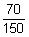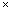100 for electronics
Percentage decrease in 1997-98 for XYZ
Engineering College =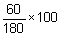for electronics
Difference =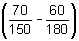100

= 13% (approx.)

Test Level 3: Bar Graph - Question 16

Directions: Answer the question based on the information given below.
The following chart shows the runs scored by Dravid in the year 2006-07.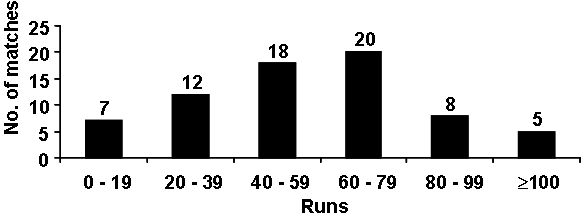Best = Highest score in any match
Average = Total runs scored/Number of outs

Q. If Dravid was out in all the innings he played, what is the maximum possible average can he have? (If his best is 120)

Detailed Solution for Test Level 3: Bar Graph - Question 16

Average =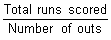, it will become maximum if total runs scored is maximum.
The maximum possible runs he can score is19 + 1239 + 1859 + 2079 + 899 + 5120 = 4635 (Since best is 120).
Total number of outs = 7 + 12 + 18 + 20 + 8 + 5 = 70.
So, Average =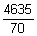= 66.21 ≈ 66.

Test Level 3: Bar Graph - Question 17

Directions: Answer the question based on the information given below.
The following chart shows the runs scored by Dravid in the year 2006-07.Q. Dravid scored 4000 runs in total during 2006-07. If his score was different in each match, what could be his best score?

Detailed Solution for Test Level 3: Bar Graph - Question 17

The score will be the highest if he scored the least possible scores in all the matches, except the match in which he scored the best.
Since he scored between 0 and 19 in 7 matches, the least possible different scores are 0, 1, 2, 3, 4, 5, 6.
Similarly for 12 matches, he scored between 20 and 39, i.e. 20, 21, 22, …, 31.
So, the possible minimum value of total runs (except the best) is
= (0 + 1 + ... + 6) + (20 + 21 + ... + 31) + (40 + 41 + ... + 57) + (60 + 61 + ... + 79) + (80 + 81 + ... + 87) + 100 + 101 + 102 + 103
=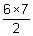+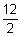(20 + 31) +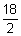(40 + 57) +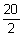(60 + 79) +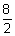(80 + 87) + 100 + 101 + 102 + 103 = 3664
The best score would be 4000 - 3664 = 336

Test Level 3: Bar Graph - Question 18

Directions: Study the following bar graph carefully and answer the question that follows.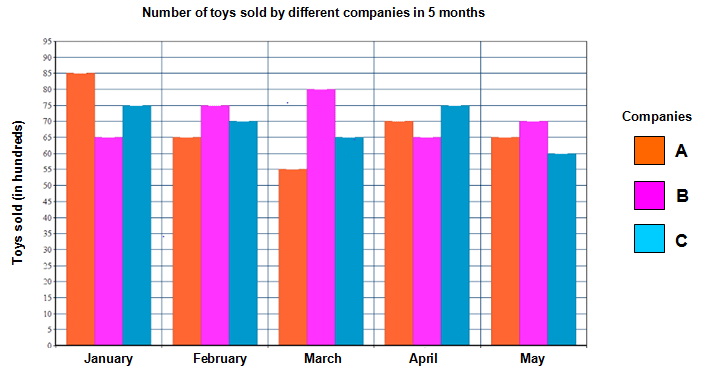Q. If the average cost of toys of company A in January was Rs. 112 and the average cost of toys of company B in the same month was Rs. 106, then find the difference between the total costs of toys of companies A and B sold in January.

Detailed Solution for Test Level 3: Bar Graph - Question 18

Total cost of toys of company A sold in January = Rs. (112 x 85) hundred= Rs. 9520 hundred = Rs. 9,52,000
Total cost of toys of company B sold in January = Rs. (106 x 65) hundred= Rs. 6890 hundred = Rs. 6,89,000
Required difference = Rs. (9,52,000 - 6,89,000) = Rs. 2,63,000

Test Level 3: Bar Graph - Question 19

Directions: Study the following bar graph carefully and answer the question that follows.Q. In February, the total sales by company A, B and C was 210 (in hundreds). In which month were the sales of all the companies in total minimum?

Detailed Solution for Test Level 3: Bar Graph - Question 19

The total sales by companies A, B and C in different months are as follows.
January = 85 + 65 + 75 = 225 (in hundreds)
February = 65 + 75 + 70 = 210 (in hundreds)
March = 55 + 80 + 65 = 200 (in hundreds)
April = 70 + 65 + 75 = 210 (in hundreds)
May = 65 + 70 + 60 = 195 (in hundreds)
Clearly, the sales were minimum in May.

Test Level 3: Bar Graph - Question 20

Directions: Study the following chart carefully and answer the question given below: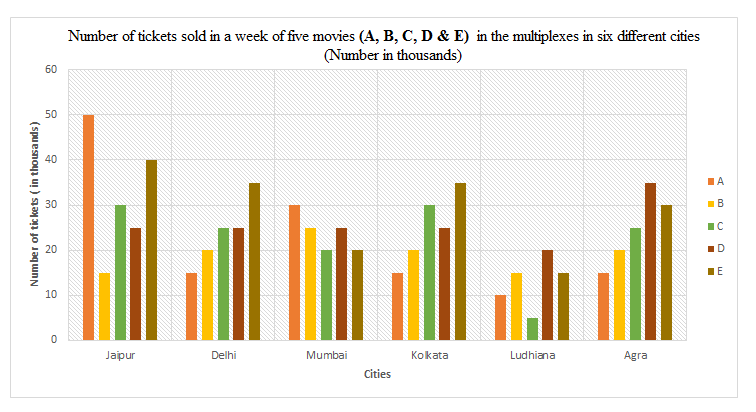Q. In which city was the total number of tickets of all the five movies together sold the minimum?

Detailed Solution for Test Level 3: Bar Graph - Question 20

Total number of tickets of all movies sold in Jaipur = 50 + 15 + 30 + 25 + 40 = 160
Total number of tickets of all movies sold in Delhi = 15 + 20 + 25 + 25 + 35 = 120
Total number of tickets of all movies sold in Mumbai = 30 + 25 + 20 + 25 + 20 = 120
Total number of tickets of all movies sold in Kolkata = 15 + 20 + 30 + 25 + 35 = 125
Total number of tickets of all movies sold in Ludhiana = 10 + 15 + 5 + 20 + 15 = 65
Total number of tickets of all movies sold in Agra = 15 + 20 + 25 + 35 + 30 = 125
The total number of tickets sold of all five movies together was the minimum in Ludhiana.

## Level-wise Tests for CAT

5 docs|272 tests
 Use Code STAYHOME200 and get INR 200 additional OFF Use Coupon Code
Information about Test Level 3: Bar Graph Page
In this test you can find the Exam questions for Test Level 3: Bar Graph solved & explained in the simplest way possible. Besides giving Questions and answers for Test Level 3: Bar Graph, EduRev gives you an ample number of Online tests for practice

## Level-wise Tests for CAT

5 docs|272 tests

### How to Prepare for CAT

Read our guide to prepare for CAT which is created by Toppers & the best Teachers